Latest Banking jobs   »

# Reasoning Ability Quiz For IBPS Clerk Prelims 2022- 6th July

Directions (1-3): Study the following information carefully and answer the questions accordingly.
Manisha starts walking in west direction from point C and walks 16m to reach point D. From point D, she walks 34m in north direction to reach point E. Then she walks 16m in west direction and reaches point F. From point F, she takes a right turn and walks 10m to reach point G.

Q1. Point G is in which direction with respect to point D?
(a) South
(b) North-East
(c) North-West
(d) West
(e) None of these

Q2. What is the shortest distance between points E and G?
(a) 12m
(b) 5√79m
(c) 2√89m
(d) 9m
(e) None of these

Q3. Point C is in which direction with respect to point F?
(a) South
(b) South-East
(c) North-West
(d) West
(e) None of these

Directions (4-6): Study the following information carefully and answer the questions accordingly.
Point O is 6m north of point S. Point G is 4m south of point K and 6m west of point F. Point S is 6m west of point X. Point K is 5m east of point O.

Q4. Point X is in which direction with respect to point O?
(a) North
(b) South-East
(c) North-West
(d) East
(e) Can’t be determined

Q5. Point K is in which direction with respect to point S?
(a) East
(b) West
(c) North-East
(d) South-West
(e) None of these

Q6. Point F is in which direction with respect to point X?
(a) South – East
(b) West
(c) North-East
(d) South-West
(e) None of these

Directions (7-9): Study the following information carefully and answer the questions accordingly.
Point C is 16m south of point D. Point G is 10m north of point H. Point G is 20m west of point F, which is 24m north of point E. Point I is 28m south of point J. Point E is 40m east of point D. Point I is 48m west of point H.

Q7. Point E is in which direction with respect to point I?
(a) East
(b) South
(c) North-West
(d) South-East
(e) Can’t be determined

Q8. What is the shortest distance between the points D and H?
(a) 30m
(b) 3√129m
(c) 2√149m
(d) 35m
(e) None of these

Q9. Point G is in which direction with respect to point C?
(a) North-West
(b) West
(c) South-West
(d) North-East
(e) None of these

Directions (10-12): Study the following information carefully and answer the questions accordingly.
Point J is 15m south of point V. Point L is 10m north of point H which is 15m east of point J. Point G is 5m south of point T which is 20m east of point V.

Q10. What is the shortest distance between points L and G?
(a) 10m
(b) 15m
(c) 5m
(d) 12m
(e) None of these

Q11. Four among the following five pair are similar in a certain way and forms a group. Which among the following pair does not belong to the group?
(a) J, L
(b) H, G
(c) L, T
(d) J, T
(e) H, V

Q12. Point T is in which direction with respect to point J?
(a) North-West
(b) West
(c) South-West
(d) North-East
(e) None of these

Directions (13-15): Study the following information carefully and answer the questions accordingly.
Point Z is 15m west of point X. Point Y is 15m west of point V. Point S is 20m south of point T. Point X is 25m north of point W which is 15m east of point R. Point S is 10m west of point R which is 20m north of point V.

Q13. Point V is in which direction with respect to point Z?
(a) South
(b) North-East
(c) North-West
(d) West
(e) None of these

Q14. Point W is in which direction with respect to point S?
(a) South
(b) North-West
(c) East
(d) North
(e) None of these

Q15. Point Y is in which direction with respect to point X?
(a) South
(b) South-West
(c) East
(d) North
(e) None of these

Solutions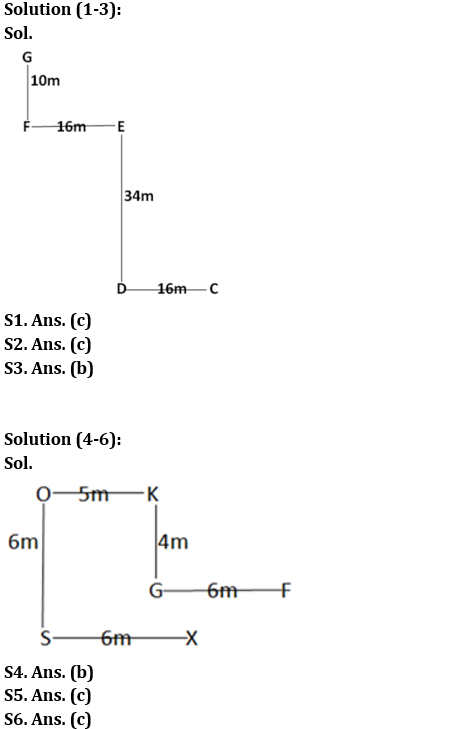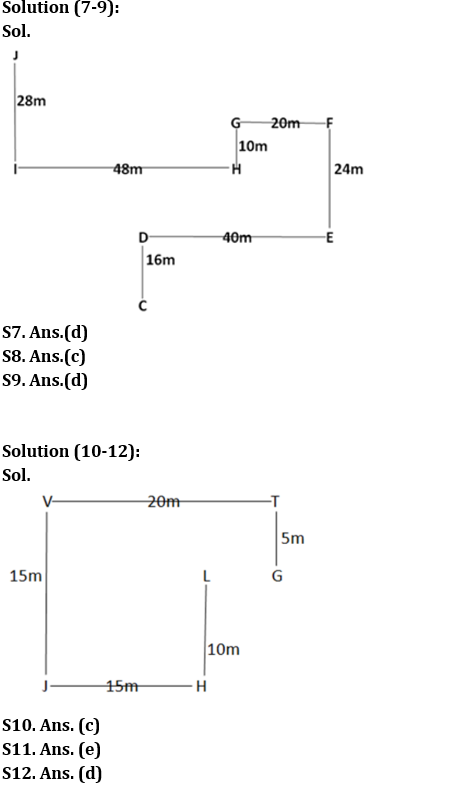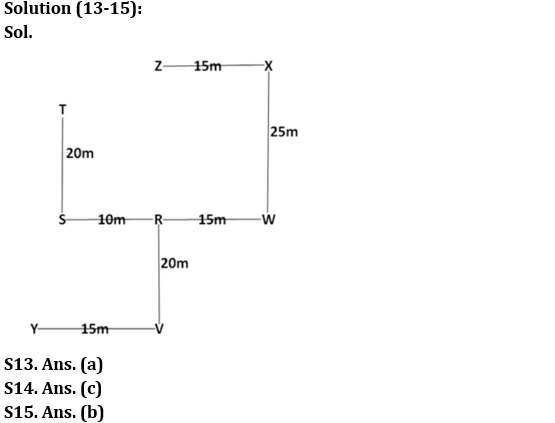#### Congratulations!Incorrect details? Fill the form again here

•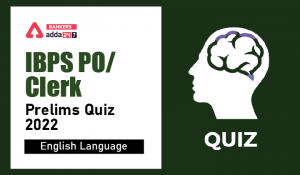English Quizzes For IBPS Clerk/PO Prelim...
•English Quizzes For IBPS Clerk/PO Prelim...
•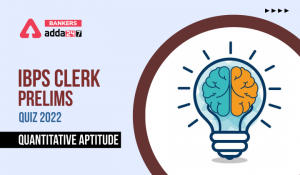Quantitative Aptitude Quiz For IBPS Cler...
•Quantitative Aptitude Quiz For IBPS Cler...
•Reasoning Ability Quiz For IBPS Clerk/PO...
•Quantitative Aptitude Quiz For IBPS Cler...